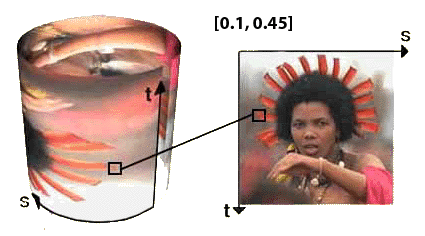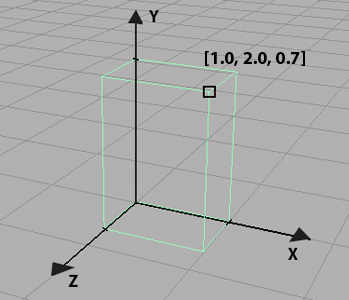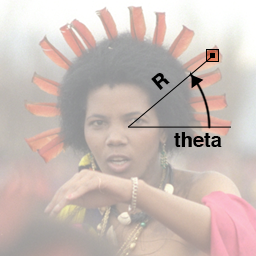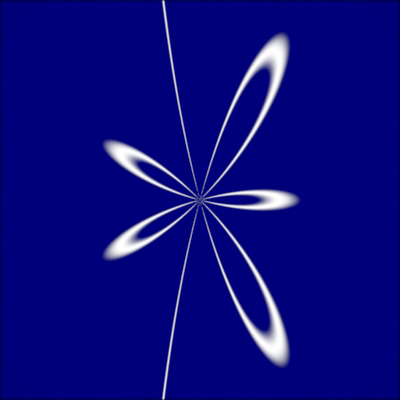### OSL2D Polar Coordinates

#### Introduction

This tutorial looks at the basics of creating patterns defined within a 2D polar coordinate system. Using polar coordinates is somewhat unusual because the majority of effects applied by shaders generally depend on the direct use of 2D 'st' texture coordinates and/or the 3D coordinates of points, vectors and normals such as the global variables `P, I` and `N`. Before looking at the use of a 2D polar coordinate system this tutorial clarifies what are generically referred to as `Cartesian` coodinates.

##### 2D Cartesian Coordinates

Locations within a 2D cartesian coordinate systems are measured relative to two axes that are perpendicular to each other. For example, figure 1 shows the coordinates (`0.1, 0.45`) of a point within a 'st' space. Although 'st' texture space uses two perpendicular axes it does not mean they must be planar. For example, 'st' texture space can be "drapped" around or over a curved surface.Figure 1

##### 3D Cartesian Coordinates

Figure 2 shows the coordinates (`1.0, 2.0, 0.7`) of a point within a 3D (xyz) cartesian coordinate space.Figure 2

##### 2D Polar Coordinates

Figure 3 illustrates how the location of a point, within a polar coordinate system, is specified by distance (`R`) and angle (`theta`). In the open shading language angles are measured in radians not degrees.Figure 3

Relative to the center of the 1 x 1 polygon, shown in figure 3, the coordinates of the hilited box are,
`R` = `0.4`
`theta` = `0.698` (ie. 40 degrees)
Since neither `R` or `theta` are global variables their values must be calculated by converting the 'st' (Cartesian) texture coordinates to their polar equivalents - listing 1.

Listing 1

 ```void polar2D(float x, float y, float xcenter, float ycenter, output float R, output float theta) { float xdiff = x - xcenter, ydiff = y - ycenter; R = sqrt(xdiff * xdiff + ydiff * ydiff); theta = atan2(ydiff, xdiff); // -PI to PI radians theta = theta + M_2PI; // 0 to 2PI radians } ```

#### Drawing Curves

The School of Mathematics and Statistics at the University of St.Andrews, Scotland maintains a web site of "Famous Curves". Many of the curves are defined by formula that require the use of polar coordinates. For example, figure 4 uses the polar description of a curve called the "Trisectrix of Maclaurin"Figure 4 - output of the Maclaurin shader

Listing 2 provides the code for the shader that produced the pattern shown above.

Listing 2 - maclaurin.osl

 ```void polar2D(float x, float y, float xcenter, float ycenter, output float R, output float theta) { float xdiff = x - xcenter, ydiff = y - ycenter; R = sqrt(xdiff * xdiff + ydiff * ydiff); theta = atan2(ydiff, xdiff); // -PI to PI radians theta = theta + M_2PI; // 0 to 2PI radians } shader maclaurin(float sc = 10 [[string help = "Scaling factor", float min = 0.01, float max = 20]], float a = 2 [[string help = "Scaling coefficient", float min = 0, float max = 10]], float b = 6 [[string help = "Lobes coefficient", float min = 0, float max = 10]], float stroke = 0.03 [[string help = "Stroke thickness", float min = 0, float max = 1]], float blur = 0.5 [[string help = "Stroke softness", float min = 0, float max = 1]], float s_center = 0.5 [[string help = "Center in 's'", float min = -2, float max = 2]], float t_center = 0.5 [[string help = "Center in 't'", float min = -2, float max = 2]], color bakcolor = color(0,0,0.2) [[string help = "Background color"]], color patcolor = 1 [[string help = "Stroke color"]], float s = 0 [[int lockgeom = 0]], float t = 0 [[int lockgeom = 0]], output color resultRGB = 0) { float R, theta; float ss = s * sc; float tt = t * sc; // Convert the s,t location of the shading point // to polar coordinates. polar2D(ss, tt, 0.5 * sc, 0.5 * sc, R, theta); // Use the MacLaurin formula float r = a * cos(b * theta)/cos(theta); float blend = smoothstep(abs(R - r) - blur, abs(R - r), stroke); resultRGB = mix(bakcolor, patcolor, blend); } ```